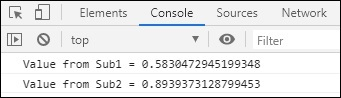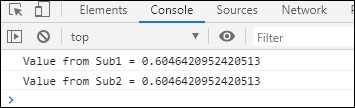# RxJS - Multicasting Operator multicast

A multicast operator shares the single subscription created with other subscribers. The params that multicast takes in is a subject or a factory method that returns a ConnectableObservable that has to connect() method. To subscribe connect() method has to be called.

## Syntax

```multicast(subjectOrSubjectFactory: Subject): OperatorFunction
```

## Params

subjectOrSubjectFactory: the parameter passed to multicast is a subject or factory method that returns a subject.

Before we get into the working of a multicast() operator, let us first understand how the multicast() operator is helpful.

Consider following example of a simple observable with subscription −

## Example

```import { Observable } from 'rxjs';

var observable = new Observable(function subscribe(subscriber) {
try {
subscriber.next(Math.random());
} catch (e) {
subscriber.error(e);
}
});
const subscribe_one = observable.subscribe(val => console.log(
"Value from Sub1 = "+val)
);
const subscribe_two = observable.subscribe(val => console.log(
"Value from Sub2 = "+val)
);
```

## OutputIf you see the output the values for Sub1 and Sub2 are different. This is because when the subscriber gets called the observable restarts and gives the fresh value available. But we need the subscribers getting called to have the same value.

Here, we have multicast() operator to help us with it.

## Example

```import { Observable,Subject } from 'rxjs';
import { take, multicast, mapTo } from 'rxjs/operators';

var observable = new Observable(function subscribe(subscriber) {
try {
subscriber.next(Math.random());
} catch (e) {
subscriber.error(e);
}
});
const multi_op = observable.pipe(multicast(() => new Subject()));
const subscribe_one = multi_op.subscribe(
x => console.log("Value from Sub1 = "+x)
);
const subscribe_two = multi_op.subscribe(
x => console.log("Value from Sub2 = "+x)
);
multi_op.connect();
```

If you now see the same value is shared between the subscribers that are called.

## Output